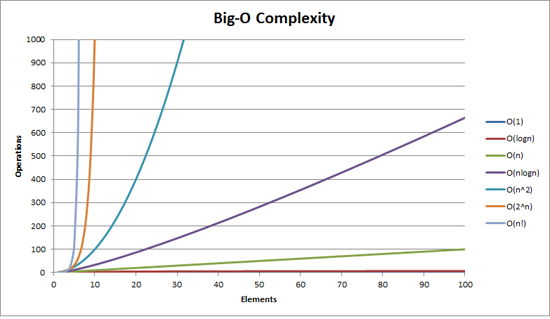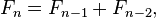## Data types and data structures

In JavaScript, there are 6 primitive data types, a primitive (primitive value, primitive data type) is data that is not an object and has no methods.

### Primitives

#### Boolean

In computer science, a boolean is a logical data type that can have only the values true or false.

#### Null

In computer science, a null value represents a reference that points, generally intentionally, to a nonexistent or invalid object or address.

#### Undefined

A primitive value automatically assigned to variables that have just been declared or to formal arguments for which there are no actual arguments.

#### Number

In JavaScript, Number is a numeric data type in the double-precision 64-bit floating point format (IEEE 754). In other programming languages different numeric types can exist, for examples: Integers, Floats, Doubles, or Bignums.

#### String

In any computer programming language, a string is a sequence of characters used to represent text.

#### Symbol (new in ECMAScript 6)

A Symbol is a unique and immutable primitive value and may be used as the key of an Object property.

``````const MY_KEY = Symbol();
let obj = {};

obj[MY_KEY] = 123;
console.log(obj[MY_KEY]); // 123
``````

### Object

Object refers to a data structure containing data and instructions for working with the data. Objects sometimes refer to real-world things like a user for instance:

``````var user = {
"first_name" : "Julien",
"last_name" : "Renaux"
}

console.log("My name is " + user["first_name"] + " " + user["last_name"]); // My name is Julien Renaux
``````

## Algorithm time complexity (Big O)

Complexity is express using big-O notation. For a problem of size N:

• a constant-time method is "order 1": O(1)
• a linear-time method is "order N": O(N)
• a quadratic-time method is "order N squared": O(N2)### For loop: O(N)

The loop executes N times, so the sequence of statements also executes N times. Since we assume the statements are O(1), the total time for the for loop is N * O(1), which is O(N) overall.

``````for (var i = 0; i < N.length; i++) {

}
``````

### Several For loops: O(max(N,M))

``````for (var i = 0; i < N.length; i++) {

}

for (var j = 0; j < M.length; j++) {

}
``````

### Nested For loops: O(N * M)

The outer loop executes N times. Every time the outer loop executes, the inner loop executes M times. As a result, the statements in the inner loop execute a total of N * M times. If N equal M then the complexity is O(N^2).

``````for (var i = 0; i < N.length; i++) {
for (var j = 0; j < M.length; j++) {

}
}
``````

### Sorting: O(N*log(N))

Typical serial sorting algorithms good behavior is O(N*log(N))

``````N.sort(function(a, b){
return a - b;
})
``````

## Classic Algorithms

What interviewers seek is to see if you can logically resolve some of the basic problems known to computer science. The following algorithms will be as clear as they can be so you can understand the logic. It is not about algorithm elegance nor performance.

### Binary search

Binary search is one of the fundamental algorithms in computer science. In its simplest form, binary search is used to quickly find a value in a sorted sequence. `git bisect` uses it to quickly find the commit that introduced a bug.

``````function binarySearch(haystack, needle) {
var lo = 0,
hi = haystack.length - 1,
mid;
while (lo <= hi) {
mid = Math.floor((lo + hi) / 2);
if (haystack[mid] < needle) {
lo = mid + 1;
} else if (haystack[mid] > needle) {
hi = mid - 1;
} else {
return mid;
}
}
return -1;
}
``````
``````// Unsorted list
var list = [80, 9, 700, 40, 1, 5, 200];

// Now sorted
list.sort(function(a,b){
return a - b;
});

// In which position is the number 200? Response: 5
binarySearch(list, 200);
``````

Complexity: Since each comparison binary search uses halves the search space, we can assert and easily prove that binary search will never use more than O(log N) comparisons to find the target value.

### Prime number

``````function isPrime(n){
var divisor = 2;

while (n > divisor){
if(n % divisor == 0){
return false;
}
else
divisor++;
}
return true;
}
``````
``````// Response: true
isPrime(137);

// Response: false
isPrime(237);
``````

### Fibonacci

In mathematics, the Fibonacci numbers or Fibonacci sequence are the numbers in the following integer sequence: 0 1, 1, 2, 3, 5, 8, 13, 21, 34, 55, 89, 144 …

It is defined by the recurrence relation:#### Code Recursive

The below algorithm performs is elegant but not efficient when the sequence grows exponentially, we get an inefficient solution.

``````function fibonacci(n){
if(n < 2) return n;
return fibonacci(n-1) + fibonacci (n-2);
}
``````
``````fibonacci(2); // Response: 1
fibonacci(3); // Response: 2
fibonacci(4); // Response: 3
fibonacci(5); // Response: 5
``````

Complexity: O(2^n) Why?

#### Code dynamic programming

We can calculate the values F0, F1, … , Fn based on the previously calculated numbers (it is sufficient to remember only the last two values).

``````function fibonacci(n){
var fiboSequence = [0, 1];

if (n < 2) return n;

for (var i = 2; i <=n; i++ ){
fiboSequence[i] = fiboSequence[i-1] + fiboSequence[i-2];
}

return fiboSequence[n];
}
``````
``````fibonacci(2); // Response: 1
fibonacci(3); // Response: 2
fibonacci(4); // Response: 3
fibonacci(5); // Response: 5
``````

Complexity: The time complexity of the above algorithm is O(n).

### Going further

Once those basic algorithms in mind if you want to go further I suggest you test yourself using Codility’s great challenges.

Some of the solutions in JavaScript are available here: Codility efficient algorithm solutions in JavaScript

## Design Patterns

Before an interview you need to know some of the most known design patterns and anti patterns. To do so I highly recommend reading Addy Osmani’s book “Learning JavaScript Design Patterns”. It is available for free http://addyosmani.com/resources/essentialjsdesignpatterns/book under http://creativecommons.org/licenses/by-nc-nd/3.0/.

### Creational

Creational design patterns are design patterns that deal with object creation mechanisms, trying to create objects in a manner suitable to the situation.

Some of the patterns that fall under this category are: Constructor, Factory, Abstract, Prototype, Singleton and Builder.

#### Factory

The Factory pattern is another creational pattern concerned with the notion of creating objects. Where it differs from the other patterns in its category is that it doesn’t explicitly require us use a constructor. Instead, a Factory can provide a generic interface for creating objects, where we can specify the type of factory object we wish to be created.

``````// A constructor for defining new cars
function Car(options) {
this.doors = options.doors || 4;
this.state = options.state || "brand new";
this.color = options.color || "silver";

}

// A constructor for defining new trucks
function Truck(options) {
this.state = options.state || "used";
this.wheelSize = options.wheelSize || "large";
this.color = options.color || "blue";
}

// Define a skeleton vehicle factory
function VehicleFactory() {}

// Define the prototypes and utilities for this factory

// Our default vehicleClass is Car
VehicleFactory.prototype.vehicleClass = Car;

// Our Factory method for creating new Vehicle instances
VehicleFactory.prototype.createVehicle = function(options) {

switch (options.vehicleType) {
case "car":
this.vehicleClass = Car;
break;
case "truck":
this.vehicleClass = Truck;
break;
//defaults to VehicleFactory.prototype.vehicleClass (Car)
}

return new this.vehicleClass(options);
};

// Create an instance of our factory that makes cars
var carFactory = new VehicleFactory();
var car = carFactory.createVehicle({
vehicleType: "car",
color: "yellow",
doors: 6
});

// Test to confirm our car was created using the vehicleClass/prototype Car

// Outputs: true
console.log(car instanceof Car);
``````

#### Prototype

We can think of the prototype pattern as being based on prototypal inheritance where we create objects which act as prototypes for other objects. The prototype object itself is effectively used as a blueprint for each object the constructor creates. If the prototype of the constructor function used contains a property called name for example (as per the code sample lower down), then each object created by that same constructor will also have this same property.

``````var myCar = {

name: "Ford Escort",

drive: function() {
console.log("Weeee. I'm driving!");
},

panic: function() {
console.log("Wait. How do you stop this thing?");
}

};

// Use Object.create to instantiate a new car
var yourCar = Object.create(myCar);

// Now we can see that one is a prototype of the other
console.log(yourCar.name);
``````

#### Singleton

The Singleton pattern is thus known because it restricts instantiation of a class to a single object. Classically, the Singleton pattern can be implemented by creating a class with a method that creates a new instance of the class if one doesn’t exist. In the event of an instance already existing, it simply returns a reference to that object.

``````var mySingleton = (function() {
// Instance stores a reference to the Singleton
var instance;

function init() {
// Private methods and variables
function privateMethod() {
console.log("I am private");
}

var privateVariable = "Im also private";

var privateRandomNumber = Math.random();

return {
// Public methods and variables
publicMethod: function() {
console.log("The public can see me!");
},
publicProperty: "I am also public",
getRandomNumber: function() {
return privateRandomNumber;
}
};
};
return {
// Get the Singleton instance if one exists
// or create one if it doesn't
getInstance: function() {
if (!instance) {
instance = init();
}
return instance;
}
};
})();

var singleA = mySingleton.getInstance();
var singleB = mySingleton.getInstance();
console.log(singleA === singleB); // true
``````

### Structural

Structural design patterns are design patterns that ease the design by identifying a simple way to realize relationships between entities.

Patterns that fall under this category include: Decorator, Facade, Flyweight, Adapter and Proxy.

#### Decorator

Decorators are a structural design pattern that aim to promote code re-use. Similar to Mixins, they can be considered another viable alternative to object sub-classing.

Classically, Decorators offered the ability to add behaviour to existing classes in a system dynamically.

``````function Vehicle(vehicleType) {
this.vehicleType = vehicleType || "car";
this.model = "default";
}

// Lets create a new instance of vehicle, to be decorated
var truck = new Vehicle("truck");

// New functionality we're decorating vehicle with
truck.setModel = function(modelName) {
this.model = modelName;
};

truck.setColor = function(color) {
this.color = color;
};

// Test the value setters and value assignment works correctly
truck.setModel("CAT");
truck.setColor("blue");

console.log(truck);

// Outputs:
// vehicle:truck, model:CAT, color: blue

// Demonstrate "vehicle" is still unaltered
var secondInstance = new Vehicle("car");
console.log(secondInstance);
``````

### Behavior

Behavioral design patterns are design patterns that identify common communication patterns between objects and realize these patterns. By doing so, these patterns increase flexibility in carrying out this communication.

Some behavioral patterns include: Iterator, Mediator, Observer and Visitor.

#### Iterator

The Iterator is a design pattern where iterators (objects that allow us to traverse through all the elements of a collection) access the elements of an aggregate object sequentially without needing to expose its underlying form.

Iterators encapsulate the internal structure of how that particular iteration occurs. In the case of jQuery’s jQuery.fn.each() iterator, we are actually able to use the underlying code behind jQuery.each() to iterate through a collection, without needing to see or understand the code working behind the scenes providing this capability.

``````\$.each(["john", "dave", "rick", "julien"], function(index, value) {
console.log(index + ": " + value);
});
``````

Here we can see the code for `jQuery.fn.each()`:

``````// Execute a callback for every element in the matched set.
each: function(callback, args) {
return jQuery.each(this, callback, args);
}
``````

Followed by a simplified version of the code behind `jQuery.each()`

``````each: function(object, callback, args) {
var name, i = 0,
length = object.length,
isObj = length === undefined || jQuery.isFunction(object);

if (isObj) {
for (name in object) {
if (callback.call(object[name], name, object[name]) === false) {
break;
}
}
} else {
for (; i < length;) {
if (callback.call(object[i], i, object[i++]) === false) {
break;
}
}
}
return object;
};
``````